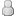• 0 Votes - 0 Average
• 1
• 2
• 3
• 4
• 5
 Shares and growth rate
03-11-2010, 01:16 AM
Post: #1
 hinanifafJunior MemberPosts: 35 Joined: Aug 2009 Reputation: 0
Shares and growth rate
Hi,
The dividend per share of T has grown from 3.50 to 10.50 over the past ten years. The share is currently selling for 75.00
What will be the growth rate and the required rate of return of T. share?

Thanks
Flora
03-15-2010, 05:27 AM
Post: #2
 Muhammad AmirPosting FreakPosts: 782 Joined: Dec 2006 Reputation: 0

<b>a) GROWTH RATE-</b>

Growth rate of dividends (for above mentioned scenario) can be determined by using Historic Pattern method.

<b>Dividend Growth = [{ (Dn/Do)^(1/n) } - 1]</b>

Where,

Dn = Most recent dividend
Do = Most earliest dividend
n = Number of years
^ = sign of "power"

Dividend Growth = { (10.5/3.5)^(1/10) } - 1

Dividend Growth = 11.61%

<b>b) RATE OF RETURN-</b>

For determination of required rate of return we can use Gordon's dividend growth model. However, this model has its own limitations and assumptions that I will mention at the end of solution.

<b>Market Value of Share = [{Dividend * (1+g)}/(Re - g)]</b>

Where,

g = dividend growth rate (using above solution 11.61% or 0.1161)
Re = Rate of return

75 = [{10.5 * (1+0.1161)}/(Re - 0.1161)]

75 = [{11.719/Re - 0.1161}]

(Re - 0.1161) = {11.719/75}

(Re - 0.1161) = 0.156254

Re = 0.156254 + 0.1161

Re = 0.2723 or 27.23%

Therefore,

<b>Rate of Return = 27.23%, and;
Growth rate = 11.61%
</b>

<b>Assumptions behind Gordon's Dividend Growth model -</b>

- Complete earnings are not distributed but retained and these are retained so that growth can occur.

- Growth rate is constant

- Next dividend is paid after 1 year

- "g (i.e. growth rate)" is less than "Re (i.e. rate of return)".
04-05-2010, 08:46 PM
Post: #3
 Qureshi Muhammad ZeeshanJunior MemberPosts: 26 Joined: Mar 2010 Reputation: 0

i m Agreed with the solution provided by Muhammad Aamir but i would like to contribute something more.

the Growth rate can also be calculated by the help of this formula which can be termed as statistical method. it is the same formula used in calculating present and future value in case of Compound Interest.
Following is the Formula
s=p*(1+i)^n
where S is the latest dividend which is 10.5 in this Question
p is the earliest dividend i-e 3.5
i= growth rate i-e to be calculated
^= sign of power(it is there in calculator)
n= n is the number of years during which dividend grows from earliest to latest i-e 10 in the above case

the other part can be solved in the same way as Muhammad Aamir did.Use Gordan's Growth Model.
04-16-2010, 04:35 PM
Post: #4
 i am a CA studentJunior MemberPosts: 10 Joined: Apr 2010 Reputation: 0

Dear,

the formula which Mr. Amir used is the methematically derived form of present value formula.

If I start with the formula you used I will derive the formula which Mr. Amir used.

S=P*(1+i)^n

S/P=(1+i)^n

multiply both sides by power 1/n.
(S/P)^1/n = {(1+i)^<font color="red">n</font id="red">*1/<font color="red">n</font id="red">}

now the "n" will be cancelled out and,

(S/P)^1/n = (1+i)^1

now power 1 means same base, hence;

(S/P)^1/n = (1+i)

{(S/P)^1/n} - 1 = i.

hence,

{(Dn/Do)^1/n - 1} = i(which is growth rate)

Regards,
04-18-2010, 06:29 AM
Post: #5
 imdad1MemberPosts: 179 Joined: Apr 2010 Reputation: 0

good yar...people here have nice concepts of Financial management...
 « Next Oldest | Next Newest »

Forum Jump:

User(s) browsing this thread: 1 Guest(s)